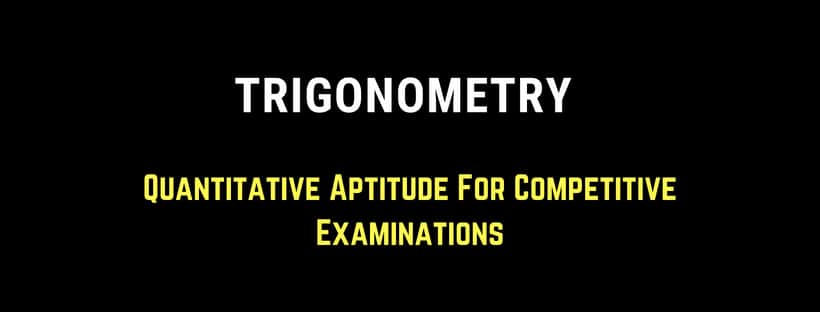Home > Aptitude and Reasoning > Trigonometry | Quantitative Aptitude For Competitive Examinations

# Trigonometry | Quantitative Aptitude For Competitive Examinations# Trigonometry Quantitative Aptitude For Competitive Examinations

Trigonometry all formulas, Practice Problems with an explanation of Solutions

Problems:

1) Find the maximum value of 24Sin

2) Find the maximum value of 5Sin2 + 2Cos2

3) The value of sin2 10 + sin2 50 + sin2 90 + ……………. + sin2 890 is ?

4) Cos x + Cos y = 2, the value of sin x + sin y is ………

5) If Sin 3A = Cos (A – 260), where 3A is an acute angle then the value of A is

6) A Circus Artist is climbing a 20 m long rope, which is tightly stretched and tied from the top of a vertical pole to the ground. Find the height of the pole, if the angle made by the rope with ground level  is 300

7) A tree breaks due to storm and the broken part bend so that the top of the tree touches the ground making an angle 300 with it. The distance between the foot of the tree to the point, where the top touches the ground is 8 m. Find the height of the tree?

and more

Learn Quantitative Aptitude For Competitive Examinations Trigonometry All Formulas Trigonometry Basics Trigonometry Problems

See Preview of EBook Recommended Quantitative Aptitude Book in Google Book Play Store